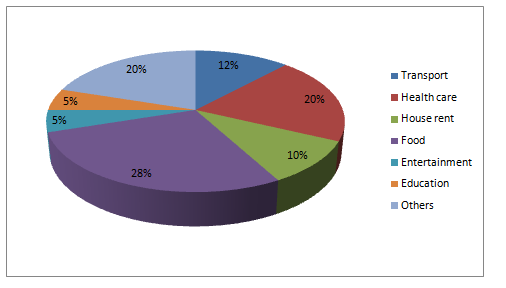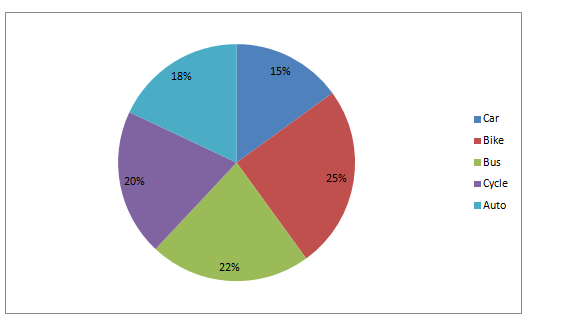# Quantitative Aptitude Questions (Data Interpretation) for SBI Clerk 2018 Day-86

Dear Readers, SBI is conducting Online preliminary Examination for the recruitment of Clerical Cadre. preliminary Examination of SBI Clerk was scheduled from June 2018. To enrich your preparation here we have providing new series of Data Interpretation – Quantitative Aptitude Questions. Candidates those who are appearing in SBI Clerk Prelims Exams can practice these Quantitative Aptitude average questions daily and make your preparation effective.

Click “Start Quiz” to attend these Questions and view Solutions

Directions (1-5): Study the following and answer the following questions.

The pie chart shows the expenditure of a person in year 2017 on various things.

Total expenditure in 2017= 2 lakhs1. What is the ratio of expenditure on Entertainment, health care and Food together to the expenditure on house rent, Education and transport together?
1. 53:27
2. 23:27
3. 27:19
4. 34:29
5. 13:25
1. What will be the average expenditure of all items except expenditure on Entertainment and others together?
1. 4 lakhs
2. 3.2 lakhs
3. 1.6 lakhs
4. 0.3 lakhs
5. 4.7 lakhs
1. If total expenditure of the person in the year 2017 is 50% of his total earnings, then expenditure on Transport is what percent of his total earnings?

a.6%

b.24%

c.14%

d.5%

e.3%

1. If person reduces his expenditure on Entertainment and other items by 10% and 20% respectively, then amount saved after reducing these expenditure will be approximately what percent of remaining total expenditure?

a.1.71%

b.4.71%

c.3.71%

d.7.71%

e.10.71%

1. If expenditure on healthcare is removed and the value is distributed equally on all other expenditure, then on which expenditure percentage increase in expenditure is Maximum?
1. Education and Entertainment
2. Food and others
3. House rent
4. Transport and food
5. Health care

Directions (6-10): Study the table and answer the following questions

The following pie chart shows distribution of different vehicles in Tamilnadu.

Total number of vehicles= 1000006) What is the central angle in the pie chart for cycles in Tamilnadu?

1. 360
2. 260
3. 620
4. 720
5. 920

7) Total number of Buses and Autos is what percentage of number of bikes in Tamilnadu?

a.60%

b.160%

c.53%

d.280%

e.100%

8) If 20% of the Bike and 10% of the cars are manufactured before the year 2000, and thus they have to be replaced by new vehicles, then what percentage of total number of vehicles were replaced?

a.3.5%

b.5.0%

c.2.0%

d.4.5%

e.6.5%

9) What is the difference between number of two wheelers and number of four wheelers in Tamilnadu considering the fact that Auto has four wheelers?

1. 10000

b.15380

c.15000

d.2830

e.6530

10) Number of Bike in Tamilnadu is what percent more than the number of cars in Tamilnadu?

a.74.67%

b.71.67%

c.52.67%

d.22.67%

e.66.67%

Expenditure on Entertainment, health care and Food together= ((5+20+28)/100)*2

= 1.06 lakhs

Similarly, Expenditure on house rent, Education and transport together= ((10+5+12)/100)*2

= 0.54 lakhs

Required ratio= 1.06: 0.54= 53:27

Total expenditure on all items except expenditure on Entertainment and others together= 2-((5+20)/100)*2

= 2-0.5

= 1.5 lakhs

Now required average= 1.5/5= 0.3 lakhs

Total earnings of a person= 2*100/50=Rs 4 lakhs

Thus required percentage=[(12*2)/(100*4)]*100= 6%

After reducing the expenditure, saved amount= (10/100*5/100+20/100*20/100)2

= (0.005+0.04)*2

= 0.09 lakhs

New expenditure= 2- 0.09=1.91 lakhs

Required percentage= 0.09/1.91*100= 4.71%

Since the expenditure on entertainment and Education is 5%, (i.e) Minimum, the percentage increase will be Maximum in these items.

Direction (6-10)

From the chart we observe that,

Then required central angle for cycles in Tamilnadu= 20/100*3600= 720

Required percentage=[(22000+18000)/25000]*100= 160%

Number of bikes have to be replaced= 20/100* 25000= 5000

Similarly, Number of cars in Tamilnadu=15000

Then, Number of cars have to be replaced= 10/100* 15000= 1500

Then Required percentage= (6500/100000)*100=6.5%

Number of Two wheelers in Tamilnadu=25000+20000= 45000

Number of four wheelers in Tamilnadu=15000+22000+18000= 55000

Required difference= 10000

Required percentage= [(25000- 15000)/15000]*100= 66.67%

Daily Practice Test Schedule | Good Luck

 Topic Daily Publishing Time Daily News Papers & Editorials 8.00 AM Current Affairs Quiz 9.00 AM Logical Reasoning 10.00 AM Quantitative Aptitude “20-20” 11.00 AM Vocabulary (Based on The Hindu) 12.00 PM Static GK Quiz 1.00 PM English Language “20-20” 2.00 PM Banking Awareness Quiz 3.00 PM Reasoning Puzzles & Seating 4.00 PM Daily Current Affairs Updates 5.00 PM Data Interpretation / Application Sums (Topic Wise) 6.00 PM Reasoning Ability “20-20” 7.00 PM English Language (New Pattern Questions) 8.00 PM General / Financial Awareness Quiz 9.00 PM